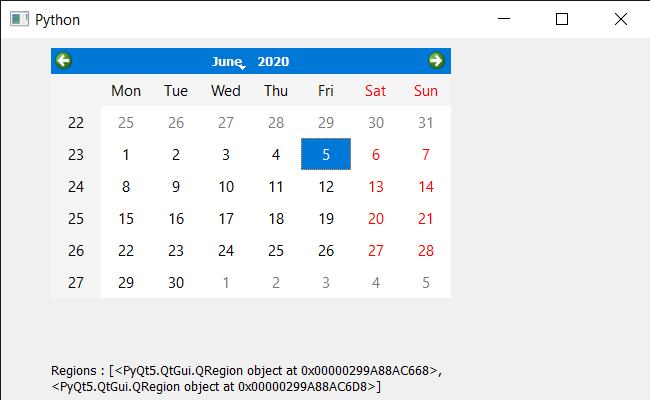# PyQt5 QCalendarWidget – Accessing Region of each children if possible

• Last Updated : 15 Dec, 2021

In this article, we will see how we get the region of all the children of QCalendarWidget. Calendar is not alone a single widget it is a mixture of lots of smaller widget which we refer as calendar’s child. There are many child such as table view, item delegate, etc, we use children method to get all the children of it. Region is the region occupied by each of children.

Note : All children don’t have rectangle as they can be a layout.

In order to do this we have to do the following :
1. Create a calendar widget
2. Get all the children of the calendar
3. Traverse the list of children
4. Try to get the visible region or children region in the try, except block and save result in another list
5. Show the list using label

Below is the implementation

## Python3

 `# importing libraries``from` `PyQt5.QtWidgets ``import` `*``from` `PyQt5 ``import` `QtCore, QtGui``from` `PyQt5.QtGui ``import` `*``from` `PyQt5.QtCore ``import` `*``import` `sys`  `class` `Window(QMainWindow):` `    ``def` `__init__(``self``):``        ``super``().__init__()` `        ``# setting title``        ``self``.setWindowTitle(``"Python "``)` `        ``# setting geometry``        ``self``.setGeometry(``100``, ``100``, ``650``, ``400``)` `        ``# calling method``        ``self``.UiComponents()` `        ``# showing all the widgets``        ``self``.show()` `    ``# method for components``    ``def` `UiComponents(``self``):` `        ``# creating a QCalendarWidget object``        ``self``.calendar ``=` `QCalendarWidget(``self``)` `        ``# setting geometry to the calendar``        ``self``.calendar.setGeometry(``50``, ``10``, ``400``, ``250``)` `        ``# creating a label``        ``label ``=` `QLabel(``self``)` `        ``# setting geometry``        ``label.setGeometry(``50``, ``280``, ``420``, ``120``)` `        ``# making it multi line``        ``label.setWordWrap(``True``)` `        ``# getting children``        ``children ``=` `self``.calendar.children()`  `        ``value ``=` `[]``        ``for` `i ``in` `children:``            ``try``:` `                ``try``:``                    ``# getting children region``                    ``region ``=` `i.childrenRegion()``                ``except``:``                    ``# getting visible region``                    ``region ``=` `i.visibleRegion()` `                ``# adding it to list``                ``value.append(region)` `            ``except``:``                ``pass` `        ``# setting text to the label``        ``label.setText(``"Regions : "` `+` `str``(value))`   `# create pyqt5 app``App ``=` `QApplication(sys.argv)` `# create the instance of our Window``window ``=` `Window()`  `# start the app``sys.exit(App.``exec``())`

Output :My Personal Notes arrow_drop_up